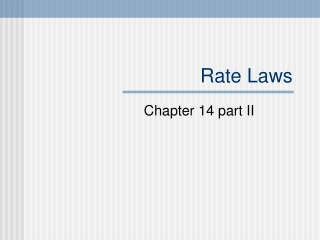DownloadDownload PresentationRate Laws

# Rate Laws

Download Presentation## Rate Laws

- - - - - - - - - - - - - - - - - - - - - - - - - - - E N D - - - - - - - - - - - - - - - - - - - - - - - - - - -
##### Presentation Transcript

1. Rate Laws Chapter 14 part II

2. Rate Laws • Chemical reactions are reversible. • So far we have only considered the forward reaction in our rates. • Eventually the rate is the difference between the forward & reverse rates. 2NO2(g) -> 2NO(g) + O2(g) 2NO(g) + O2(g) -> 2NO2(g) Rate1 = -∆[NO2]/∆t Rate2 = ∆[NO]/∆t (-∆[NO2]/∆t) -(∆[NO]/∆t

3. How to deal with that? • For our purposes, we will concentrate on reactions that in which the reverse rate will be insignificant. Specifically when the [products] is very low to zero.

4. Rate1 = -∆[NO2]/∆t Rate2 = ∆[NO]/∆t The Integrated Rate Law expresses how the concentration depends on time. Integrated Rate Law

5. Differential Rate Law • Differential rate law expresses how the rate depends on concentration. • The differential rate law is also known as just Rate Law. Rate = k[NO2]n k is the rate constant n is called the order of the reaction The order of a reaction must be determined experimentally.

6. Rate = k[NO2]n= -∆[NO2]/∆t Rate may be defined in different ways.

7. 2NO2(g) -> 2NO(g)+O2(g) In the integrated rate law, the rate can be defined using any of the species in the equation, product or reactant. However, one must be careful. In this case it takes 2 NO2 to make one O2. Defining rates

8. Defining Rate Laws • If Rate1 = -∆[NO2]/∆t = k[NO2]n • Then Rate2 = ∆[O2]/∆t = k’ [NO2]n • Since 2NO2 molecules are consumed for every O2 molecule produced, • Rate = 2 x Rate’ • Or k[NO2]n = 2 k’ [NO2]n • Therefore k = 2 x k’ • The value of the rate constant depends on how the rate is defined.

9. The value of the exponent n must be determined experimentally; it cannot be written from the balanced equation. The concentration of the products do not appear in the rate law because the reaction is being studied under conditions where the reverse reaction does not significantly contribute to the overall rate. Two important points

10. Summary • There are two types of rate laws: • Intergrated & differential • We consider rates at where the reverse reaction is insignificant so rate laws involve only [reactants]. • Because both laws for a reaction are well defined, the experimental determination of either rate law is sufficient. • Finally: • From the rate law, one may infer the mechanism of a reaction.

11. Determining the Form • First one needs to determine the form of the rate law • We need to look at how the rate changes as the concentration changes. Consider dinitrogen pentaoxide in carbon tetrachloride: 2N2O5(soln) -> 4NO2(soln) + O2(g)

12. 2N2O5(soln) -> 4NO2(soln) + O2(g)

13. Rate Laws: First Order • First determine how the rate changes as the concentration changes. • We find that as the concentration is halved, the rate is halved. • This is the definition of a First Order RXN. [N2O5] Rate M/s 0.90 M 5.4 x 10-4 0.45 2.7 x 10-4 Rate =( - ∆[N2O5]/∆t) = k[N2O5]1 = k[N2O5]

14. First Order Reactions • Rate = - ∆[A]/∆t = k[A] • Where A is the concentration of the reactant.

15. Method of Initial Rates • The initial rate is where the reaction has just started, just after t=0. • Several experiments are carried out using different concentrations and the initial rate is determined for each run. • The rates are then compared to the concentrations to see how the rate depends on the initial concentration.

16. # [NH4+]M [NO2-]M Rate M/s 0.100 0.0050 1.35x10-7 0.100 0.010 2.70x10-7 0.200 0.010 5.40x10-7 The general for of the rate law is… Rate=-∆[NH4+]/∆t =k[NH4+]n[NO2-]m We can determine the values of m & n experimentally See: Rate2/Rate1 For Example: NH4+(aq)+NO2-(aq)-> N2(g)+2H2O(l) Rate2 =2.70x10-7M/s=k(0.100M)n(0.010M)m Rate1 1.35x10-7M/s k(0.100M)n(0.005M)m

17. Determining a Rate Law # [NH4+]M [NO2-]M Rate M/s • 0.100 0.0050 1.35x10-7 • 0.100 0.010 2.70x10-7 • 0.200 0.010 5.40x10-7 Rate2 =2.70x10-7M/s=k(0.100M)n(0.010M)m Rate1 1.35x10-7M/s k(0.100M)n(0.005M)m 2 =(0.010M)m = (2.0)m m=1 (0.0050M)m

18. Since m=1 that means the rate law is first order for [NO2-] Now find the value of n. Since both n & m are 1, the rate law is written k [NH4+][NO2-] # [NH4+]M [NO2-]M Rate M/s 0.100 0.0050 1.35x10-7 0.100 0.010 2.70x10-7 0.200 0.010 5.40x10-7 NH4+(aq)+NO2-(aq)-> N2(g)+2H2O(l)

19. Since the sum of the orders in this rate law is two, n&m=2, then the rate law is said to have an overall reaction order of 2 How do you find the value for k? Chose one experiment and add in your known values to solve for k. What is k? 2.7x10-4 l/mol•s Overall Rate

20. Examples • . Calculator problem - Cyclopropane rearranges to form propene: • CH2CH2CH2 --> CH2=CHCH3 • by first-order kinetics. The rate constant is k = 2.74 x 10-3 s-1. The initial concentration of cyclopropane is 0.290 M. What will be the concentration of cyclopropane after 100 seconds? • a) 0.220 Mb) 0.760 Mc) 2.74 x 10-1 Md) 7.94 x 10-2 M